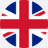# How to convert CGPA to percentage calculator for DU

Delhi University (DU) is one of the most prestigious universities in India, and employers and universities across the country widely recognize its grading system. The university uses a Cumulative Grade Point Average (CGPA) system to evaluate a student's academic performance. However, for certain purposes, such as applying for a job or pursuing higher studies, students may need to convert their CGPA into a percentage. In this article, we will discuss the process of converting DU CGPA to percentage, including the steps, formula, and tools required.

Also read: How to convert CGPA to Percentage?

## DU CGPA to Percentage Conversion:

To convert DU CGPA to percentage, students need to follow the below steps:

Step 1: Determine the CGPA obtained by the student. CGPA is calculated by taking the weighted average of all the grades obtained by the student in all the semesters/years of the academic program.

Step 2: Multiply the CGPA obtained in step 1 by 9.5. The result value will be the percentage equivalent of the CGPA.

### Formula for converting DU CGPA to Percentage:

The formula for converting DU CGPA to percentage is as follows:

Percentage of marks = 9.5 x CGPA

For example, if a student has a CGPA of 8.5, the percentage equivalent would be:

Percentage of marks = 9.5 x 8.5 = 80.75%

Therefore, the student's percentage of marks would be 80.75%.

## DU CGPA to Percentage Certificate:

DU provides a conversion certificate for students who need to convert their CGPA into a percentage. Students can obtain this certificate by submitting a request to the Controller of Examination at DU. The certificate contains the student's name, registration number, program name, CGPA, and percentage equivalent.

## DU CGPA to Percentage Calculator

Students can use a DU CGPA to percentage calculator to convert their CGPA into a percentage accurately. Several online CGPA to percentage calculators are available, which require students to enter their CGPA, and the calculator will provide the percentage equivalent. Students should ensure that they use a reliable and accurate calculator to avoid any discrepancies in their calculations.

Converting DU CGPA to percentage is an easy process requiring students to follow the formula mentioned above and the steps. Students can also obtain a conversion certificate from DU or use a CGPA to percentage calculator for convenience. Converting CGPA to percentage can be useful for students who wish to pursue higher studies or apply for jobs that require a percentage-based evaluation of their academic performance.

Related pages,

## FAQ’s:

### Q: Why do students need to convert their DU CGPA into percentage?

A: Students may need to convert their DU CGPA into percentage for various purposes, such as applying for higher studies, scholarships, or jobs that require a percentage-based evaluation of academic performance.

### Q: Is the conversion formula the same for all universities in India?

A: No, the conversion formula may vary depending on the university. Therefore, students should check with their respective universities for the correct formula.

### Q: Can a CGPA of 10 be converted to 100%?

A: No, a CGPA of 10 cannot be converted to 100% as the maximum percentage that can be obtained is 95%.

### Q: What is the minimum percentage equivalent of a CGPA of 6.0?

A: The minimum percentage equivalent of a CGPA of 6.0 is 57%.

### Q: Can students use online CGPA to percentage calculators to convert their CGPA?

A: Yes, students can use online CGPA to percentage calculators to convert their CGPA. However, they should ensure that they use a reliable and accurate calculator to avoid any discrepancies in their calculations.

### Q: Is it mandatory to obtain a conversion certificate from DU?

A: No, it is not mandatory to obtain a conversion certificate from DU. However, some institutions or employers may require students to provide a conversion certificate as proof of their academic performance. In such cases, students can obtain a certificate from DU by submitting a request to the Controller of Examination.£
GBP